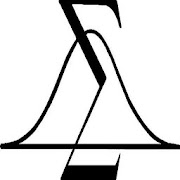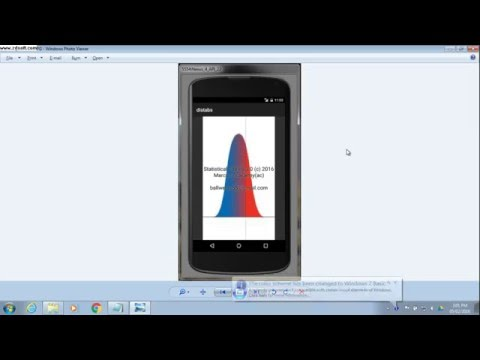# Statistical Tables 3.2Everyone
135Version 3.2 changes:

Up to date Build of APP. July of 2019.

Version 3.1 changes:

Now you can choose to show or omit background graphs on the Probability Table text views. Choose how you want it on the About Screen. Also, you can now copy the Probability Table text results from the answer in the Probability Table text views.

Version 3.0 includes:

Hypothesis Test Mean (Sigma Known, Sigma
Unknown, Proportion).
Inference Test Formulas for 2 Populations -
Means and Proportions.

Version 2.9

electronic Probability Distribution Tables.

Normal, Binomial, Poisson, T and F
Distributions. Also, invNorm and
invT calculations.

Contains calculators for Confidence
Intervals (Sigma Known, Sigma Unknown,
Proportion).

Contains calculators for Permutations
and Combinations, and Sample Size.

Updated to include:

Hypothesis Test Mean (Sigma Known, Sigma
Unknown, Proportion).
Inference Test Formulas for 2 Populations -
Means and Proportions.

Great for Intro Probability Statistics
courses, or if you just need these tables!

For both Phone and 10' tablets.

These tables give you the probabilities
associated with your test statistics and
parameters.

To use these tables, just select the
distribution you want, and put in the
test statistic and parameters, and the
APP tells you the related probability.

*************************************

1) For the Normal Distribution:

Enter your Z Score, and you get
the left, right tails and the range.

2) For the Binomial Distribution:

Enter n - number of trials,
p - probability of success,
x - number of successes

and you get the probability of
x, or the cumulative probability
for x.

3) For the Poisson Distribution:

Enter the mean, and number of
occurences, and you will get
the Probability of the number
of occurences, and the cumulative
probability.

4) For the t Distribution:

Enter t and the Degrees of Freedom
and you will get the probability
of the left and right tails, and
the two tailed probability.

5) For the f Distribution:

Enter the f statistic, and the
Degrees of Freedom for the numerator
and denominator. You will get the
probability for the right tail
(alpha for the ANOVA test).

6) For the Chi-Square Distribution:

Enter Chi-Square and the Degrees of Freedom
and you will get the probability
of the right tail.

7) Inverse Normal.

Enter the probability of the
Normal Distribution left tail,
and you get the Z statistic.

8) Inverse t

Enter the probability of the
t distribution left tail, along with
the degrees of freedom, and you will
get the t statistic.

Confidence Intervals:

Sigma Known. Uses the standard
CI formula (Using Z) to determine the range with
CI% chance of containing the true mean
of the population.

Sigma unKnown. Uses the standard
CI formula (Using t) to determine the range with
CI% chance of containing the true mean
of the population.

CI for a proportion. Uses the standard
CI formula (Using p,n,Z) to determine the range with
CI% chance of containing the true mean
of the population.

StatUtils:

Permutations and Combinations give number
of ways to select n Items out of N Items.
In Permutations Order of selected Items matters,
in Combinations it does not. Sampling is
without replacement.

Sample size n, is number needed for CI with
following parameters:

alpha - CI between 0 and 1.0
sigma - Known standard deviation.
E - desired error.

Formula is: n = (Z^2 * s^2) / E^2

Z is Z score for (1-alpha)/2

Free, but please visit this APP in
the Google Play store to Rate and
enter comments. This will help us
improve the APP over time.

Due care is taken in creating this APP.
However no warranty is intended or
implied.

Keywords: statistics statistical tables
probability distribution confidence interval
permutations combinations
Collapse

Review Policy
4.3
135 total
5
4
3
2
1

## What's New

Version 3.2 Updated:

Up to date Build of APP. July 2019

Now you can choose to show or omit background graphs in the Probability Table textviews. Choose what you want on the About Screen. Also, you can now copy the Probability Table text results from the answer on the screen.
Collapse

Updated
July 16, 2019
Size
2.5M
Installs
10,000+
Current Version
3.2
Requires Android
8.0 and up
Content Rating
Everyone
Permissions
Offered By